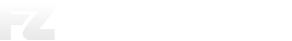# Determining Support and Resistance Levels Through Pivot Points

## Discussion started on Trading Systems

#### Upnormal12

Determining Support and Resistance Levels Through Pivot Points

Good morning friend and admin , accommodating and all of the board members of this beloved forum, please permit to open a thread that will wipe around Determining Support and Resistance Levels Through Pivot Points which we can later make as one of the references in trading.

As we know, there are so many traders who trade by making support and resistance levels as a reference to open positions, determine take profit, install SL or just want to predict how much the price level might move.

There are several ways that traders do to determine the levels, such as using Fibonnaci or you can also observe the previous price movements such as the previous highest price, the previous lowest price or there is also a price area that is often touched which is considered Support and Resistance. In general, the determination of the SnR level is considered as a subjective determination where the determination of these levels is based on visual observation and each trader has different assumptions in marking a price level that is considered as the Support and Resistance levels.

All of the methods carried out are nothing wrong, all are considered correct as long as making our trading comfortable and sure to be referred to as a tool to project the direction of price movements correctly.

What I will discuss in this thread is determining Support and Resistance (SnR) levels based on Pivot Points.

What is a pivot point? Pivot Points can generally be interpreted as a level or number obtained from the movement of the highest, lowest prices and closing prices of the previous period which will later be used as a reference in determining price levels that will be used as Support and Resistance.

How do you calculate and determine the price level used as a pivot point? I will discuss it in the next post.

#### Upnormal12

In determining the price level that will be used as a pivot point, we need to know the highest price of the previous period. I prefer to use the Daily period where we will find out the highest price, the lowest and the closing of a pair yesterday.

For example, this time I will use the EURUSD pair and find out the highest, low and closing prices on Friday June 14 2019
If colleagues use Meta Trader on PC, to find out the Highest Price (H), Lowest Price (L) and Closing Price (C) by pointing the mouse pointer on the candle we will use and at the bottom the meta trader will display the highest price level lowest and closing yesterdayAs for colleagues who use Meta Trader on android, select the OHCL setting and mark, then return to the main chart and use TF Daily then highlight the candle yesterday, then it will appear in the upper left corner of the OHCL priceof the two images that I attached then obtained:
Opening Price (O) = 1.1274
Highest price (H) = 1.1289
Lowest Price (L) = 1.1202
Closing Price (C) = 1.1208
Pivot Points (P) = ...?

Before determining Support and Resistance, we must first get a number or level of Pivot Points

Pivot Points = (H + L + C) / 3
= (1.1289 + 1.1202 + 1.1208 / 3
= 1.1233

We have obtained Pivot points at the price level of 1.1233 to be used as a reference in determining Support and Resistance / [R1] ~ [R2] ~ [R3] ~ [S1] ~ [S2] ~ [S3]

To determine Level 2 SnR based on the Pivot Points we have already obtained, I will discuss it in the next post

#### Upnormal12

Now I will try to determine the Support and Resistance levels based on the Pivot points that we have obtained, and I do the calculations manually. If you are lazy to count manually, actually many calculator applications pivot points on the Google Plya store or even indie, which can automatically display a complete pivot point with the SnR level2 on the Meta Trader PC.
Previously, I would give the Support and Resistance calculation formula first

Opening Price (Open) = O
Highest Price (High) = H
Lowest Price (Low) = L
Closing Price (Close) = C
Pivot Point = P

P = = (H + L + C) / 3
R1 = (2xP) - L
R2 = P + H - L
R3 = H + 2x (P-L)
S1 = (2xP) - H
S2 = P - H - L
S3 = L - 2x (H-P)

EURUSD position Friday June 14 2019:
O = 1.1274
H = 1.1289
L = 1.1202
C = 1.1208
P = 1.1233 (1.1289 + 1.1202 + 1.1208) / 3

R1 = (2x1.1233) - 1.1202 = 1.1264
R2 = 1.1233 + 1.1289 - 1.1202 = 1.1320
R3 = 1.1289 + 2x (1.1233 - 1.1202) = 1.1351
S1 = (2x1.1233) - 1.1289 = 1.1177
S2 = 1.1233 - 1.1289 - 1.1202 = 1.1146
S3 = 1.1202 - 2x (1.1289-1.1233) = 1.1090

[R1: 1.1264] ~ [R2: 1.1320] ~ [R3: 1.1351]
[S1: 1.1177] ~ [S2: 1.1146] ~ [S3: 1.1090]
Pivot Points: 1.1233Where to see or estimate the price will move, we will try to do it tomorrow tomorrow and we can learn and if the writing that I share is still many shortcomings I can improve.

### Members:

0 Members and 1 Guest are viewing this topic.

###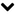Similar Topics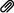###### Indicator all Pivot points

Started by SamuraiFx on MT4 / MT5 Indicators

11 Replies
2393 Views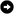June 18, 2020, 11:52:54 AM
by kisumam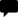###### Maximizing Technical with Pivot points

Started by Nendi71 on Technical

0 Replies
466 ViewsFebruary 03, 2019, 07:35:03 PM
by Nendi71###### Pivot Points in Forex Trading

Started by zanurreload on Forex Education

291 Replies
6507 ViewsJune 22, 2023, 04:02:19 AM
by YengocApril 20, 2019, 12:31:55 AM